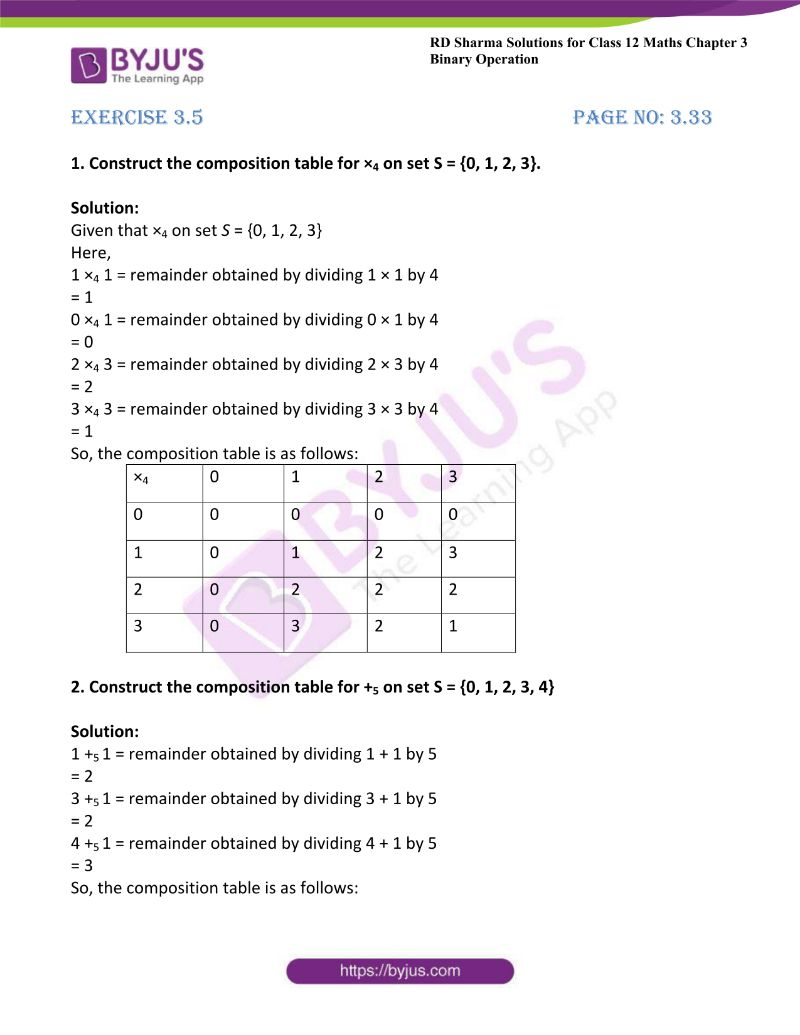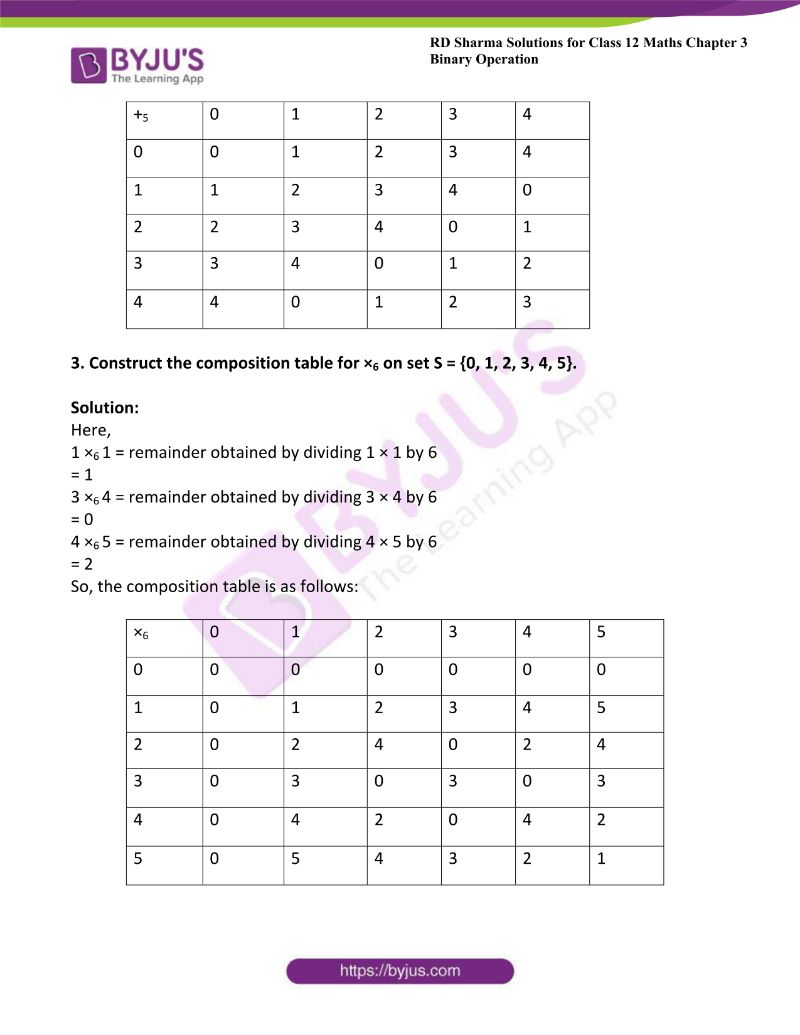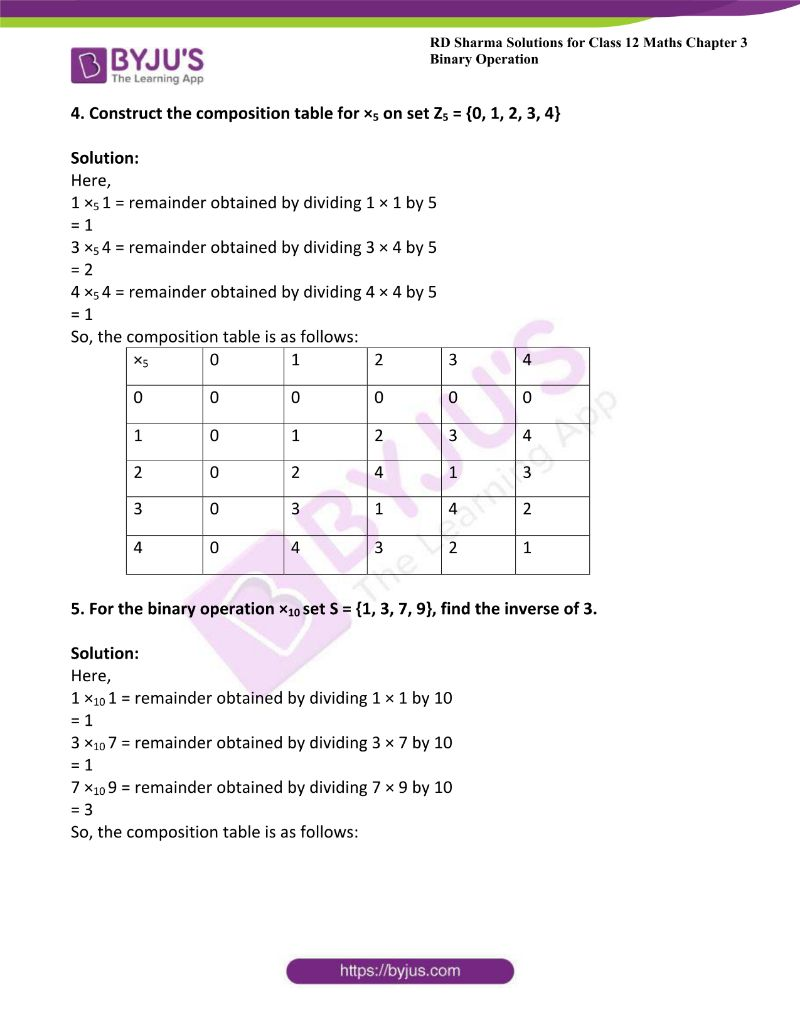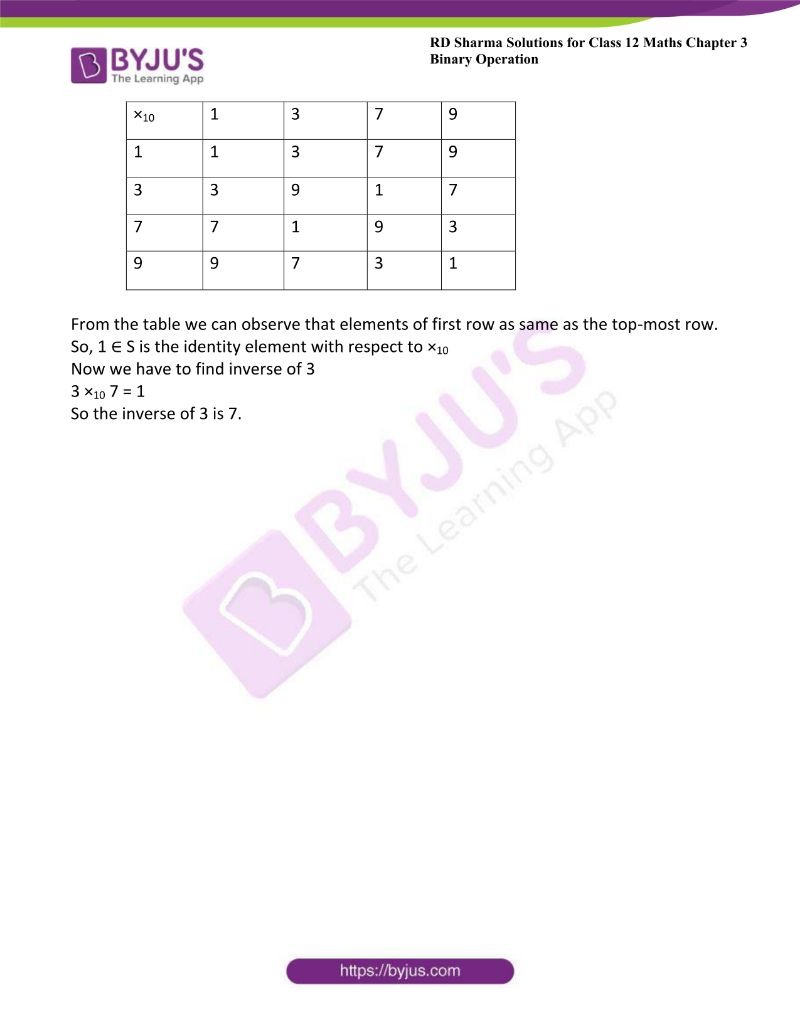# RD Sharma Solutions For Class 12 Maths Exercise 3.5 Chapter 3 Binary Operations

Composition table and multiplication modulo of binary operations is the main concept which is covered under exercise 3.5 of Chapter 3. The RD Sharma textbook contains examples before each exercise to improve problem-solving speed among students. These concepts are explained in simple language, which makes it possible even for a weak student to understand the subject better. Solutions are prepared by a set of experts at BYJU’S with the aim of helping students boost their exam preparation. In order to score well in the board exam, students can use RD Sharma Solutions Class 12 Maths Chapter 3 Binary Operations Exercise 3.5 PDF, which are available here as a reference guide.

## Download the PDF of RD Sharma Solutions For Class 12 Chapter 3 – Binary Operations Exercise 3.5### Access answers to Maths RD Sharma Solutions For Class 12 Chapter 3 – Function Exercise 3.5

1. Construct the composition table for ×4 on set S = {0, 1, 2, 3}.

Solution:

Given that ×4 on set S = {0, 1, 2, 3}

Here,

1 ×4 1 = remainder obtained by dividing 1 × 1 by 4

= 1

0 ×4 1 = remainder obtained by dividing 0 × 1 by 4

= 0

2 ×4 3 = remainder obtained by dividing 2 × 3 by 4

= 2

3 ×4 3 = remainder obtained by dividing 3 × 3 by 4

= 1

So, the composition table is as follows:

 ×4 0 1 2 3 0 0 0 0 0 1 0 1 2 3 2 0 2 2 2 3 0 3 2 1

2. Construct the composition table for +5 on set S = {0, 1, 2, 3, 4}

Solution:

1 +5 1 = remainder obtained by dividing 1 + 1 by 5

= 2

3 +5 1 = remainder obtained by dividing 3 + 1 by 5

= 2

4 +5 1 = remainder obtained by dividing 4 + 1 by 5

= 3

So, the composition table is as follows:

 +5 0 1 2 3 4 0 0 1 2 3 4 1 1 2 3 4 0 2 2 3 4 0 1 3 3 4 0 1 2 4 4 0 1 2 3

3. Construct the composition table for ×6 on set S = {0, 1, 2, 3, 4, 5}.

Solution:

Here,

1 ×6 1 = remainder obtained by dividing 1 × 1 by 6

= 1

3 ×6 4 = remainder obtained by dividing 3 × 4 by 6

= 0

4 ×6 5 = remainder obtained by dividing 4 × 5 by 6

= 2

So, the composition table is as follows:

 ×6 0 1 2 3 4 5 0 0 0 0 0 0 0 1 0 1 2 3 4 5 2 0 2 4 0 2 4 3 0 3 0 3 0 3 4 0 4 2 0 4 2 5 0 5 4 3 2 1

4. Construct the composition table for ×5 on set Z5 = {0, 1, 2, 3, 4}

Solution:

Here,

1 ×5 1 = remainder obtained by dividing 1 × 1 by 5

= 1

3 ×5 4 = remainder obtained by dividing 3 × 4 by 5

= 2

4 ×5 4 = remainder obtained by dividing 4 × 4 by 5

= 1

So, the composition table is as follows:

 ×5 0 1 2 3 4 0 0 0 0 0 0 1 0 1 2 3 4 2 0 2 4 1 3 3 0 3 1 4 2 4 0 4 3 2 1

5. For the binary operation ×10 set S = {1, 3, 7, 9}, find the inverse of 3.

Solution:

Here,

1 ×10 1 = remainder obtained by dividing 1 × 1 by 10

= 1

3 ×10 7 = remainder obtained by dividing 3 × 7 by 10

= 1

7 ×10 9 = remainder obtained by dividing 7 × 9 by 10

= 3

So, the composition table is as follows:

 ×10 1 3 7 9 1 1 3 7 9 3 3 9 1 7 7 7 1 9 3 9 9 7 3 1

From the table we can observe that elements of first row as same as the top-most row.

So, 1 ∈ S is the identity element with respect to ×10

Now we have to find inverse of 3

3 ×10 7 = 1

So the inverse of 3 is 7.